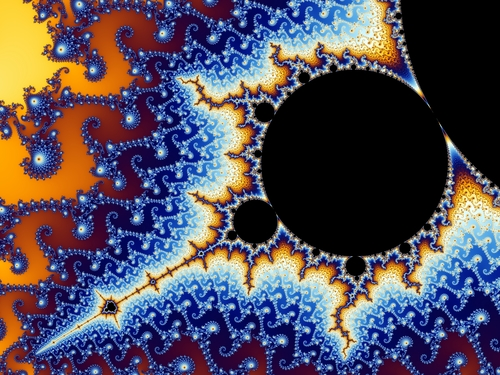Area of Triangles and Parallelograms
3 years ago
mpchale
Save
Edit
Host a game
Live GameLive
Homework
Solo Practice
Practice12 QuestionsShow answers
• Question 1
120 secondsQ. What is the area of this 5cm by 8cm rectangle?
40 square cm
40 cm
40
13
• Question 2
120 secondsQ. What is the area of the triangle shown?
44.4 mm²
8.5 mm²
8.88 mm²
17.76 mm²
• Question 3
120 secondsQ. Find the area of the parallelogram.
12 units2
10 units2
13 units2
6 units
• Question 4
120 seconds
Q. A parallelogram has a base of 35 cm and its area is 700 cm2. What is its height?
20 cm
24,500 cm
0.05 cm
2 cm
• Question 5
30 seconds
Q. What is the area of a triangle with a height of 10 m and a base of 6 m?
35 metres squared
16 metres squared
60 metres squared
30 metres squared
• Question 6
60 seconds
Q. Find the area of a triangle with a height of 4 mm and a base of 6 mm?
5 mm squared
6 mm squared
12 mm squared
24 mm squared
• Question 7
120 seconds
Q. What is the area of a square with a length of 4 cm?
16 cm
16 square cm
8 cm
8 square cm
• Question 8
300 secondsQ. Find the Area of this triangle.
(the side length is 7km)
19.8 km2
37.8 km2
18.9 km2
35 km2
• Question 9
900 secondsQ. Find the area of the parallelogram.
15.6 cm2
30.6 cm2
13.26 cm2
79.56 cm2
• Question 10
60 secondsQ. What is the area?
12 cm2
14
14 cm2
24 cm2
• Question 11
300 seconds
Q. What is the area of a parallelogram that has a base of 8.5 cm and a height of 5.2 cm?
44.2 square cm
22.2 square cm
19.2 square cm
13.7 square cm
• Question 12
120 secondsQ. Find the area of the figure.